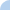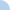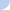# Quiz on solving a system of equations

For most questions you will be re-arranging the steps in the correct order.
Remember that a SOLUTION is a number that makes the system (both equations) true; it is also the place where the lines would intersect.

 This quiz is timed. The total time allowed for this quiz is 5 hours.Quiz Log In